Home > Electronic Tutorials > Digital Circuits Tutorial > Binary Tutorial

Digital Circuits - Electronic Tutorials

# Binary Tutorial

Humans have 10 fingers so they use the numbers 0 to 9 for counting.

Penguins have only two flippers so they can only use 0 and 1.

Brand new human and penguin cars have all zeros displayed on the odometer.After traveling 1 mile both display.So a 1 in this first column means 1 mile in both cars.

After traveling another mile, the humans display shows.However, as we saw at the start, the penguin doesn't have a 2.

So he resets the first column to zero and puts a 1 in the next column.He says to himself, "a 1 in this second column is worth 2 in decimal".

They both drive another mile. The humans car displays 3.Since the penguin has driven the same mile, he puts a 1 in the first column, which we know is worth 1 mile in both cars.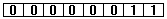They drive another mile. The humans car shows 4The penguin has to reset the first two columns to zero and poke a 1 in the third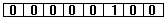He says, " a 1 in the third column is the worth 4 in the decimal system."

So we can correctly guess that a 1 in the fourth column indicate decimal 8.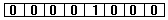and a 1 in the next is worth 16, and so on.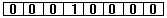So when the odometer shows 1111.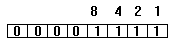the car has traveled 8+4 +2 +1 =15 decimal miles.

So adding up in penguin is

0+0=0
0+1=1
1+0=1
1+1= zero and carry 1 = 10

Write the numbers from nought to fifteen, decimal, in penguin.

Penguin counting is the same as BINARY COUNTING.

Binary is used in digital electronic systems, where they have only two values of voltage.